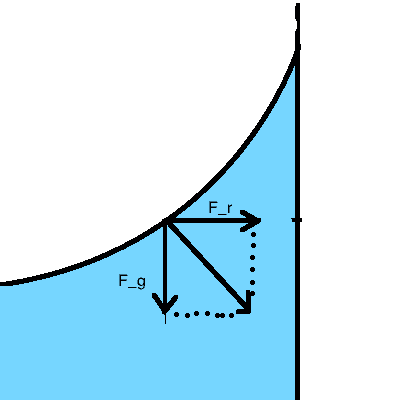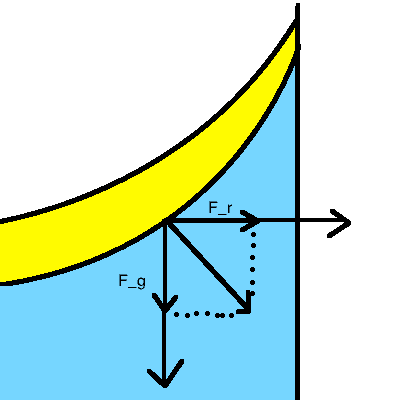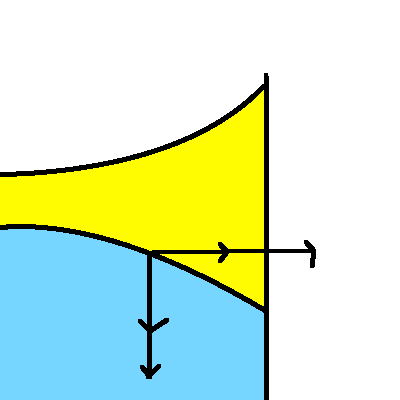# Surface between 2 rotating fluids.

## Homework Statement

Oil and water are put in a cylindrical container. They can rotate at various frequency around the rotational symmetry axis.
Consider gravitational and radial forces to find the form of the surface between the fluids.
no friction, no mixing
the surface has the form of a parabloid.
surfaces are not touching each other or the bottom of the container.

## Homework Equations

$F_r=mr\omega ^2$
$F_g=mg$

## The Attempt at a Solution

I have found a solution, but it only applies when both (oil-air ,water-oil) parabloids have the form of $y=r^2$, but observation suggests, that if the water is still and the oil rotates, that the oil-water surface is of the form $y=-r^2$

Edit:i found the upper surface by saying:
the slope(derivative of the function y(r)) is given by the fact, that the resulting force is applied orthogonal to the surface.
this gives:
$\frac{dy}{dr}=\frac{F_r}{F_g}=\frac{mr\omega ^2}{mg}=\frac{f\omega ^2}{g}$
Integrating:
$y=\frac{r^2 \omega ^2}{2g}+C$

Edit 2:
Now I thought I could simply pull of this principle like in this picture to find the surface between the two fluids, simply adding the respective forces, but this stops making sense as soon as you see the surface between water and oil having the form $y=-r^2$Once the surface turns this way, the vectors stop making sense.Last edited: